Refer to our Texas Go Math Grade 5 Answer Key Pdf to score good marks in the exams. Test yourself by practicing the problems from Texas Go Math Grade 5 Lesson 11.3 Answer Key Quadrilaterals.

Essential Question
How can you classify and compare quadrilaterals?Unlock the Problem
A seating chart for a baseball field has many four-sided figures or quadrilaterals. What types of quadrilaterals can you find in the seating chart?

There are five special types of quadrilaterals. You can classify quadrilaterals by their properties, such as parallel sides and perpendicular sides. Parallel lines are lines that are always the same distance apart. Perpendicular lines are lines that intersect to form four right angles.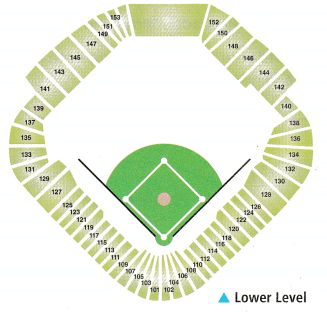Complete the sentence that describes each type of quadrilateral.

A general quadrilateral has 4 sides and 4 angles.A rectangle is a special parallelogram with 4 right angles and 4 pairs of congruent sides.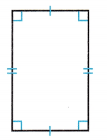A square is a special parallelogram with 4 congruent sides and 4 right angles.A parallelogram has opposite sides that are congruent and parallel.A rhombus is a special parallelogram with 2 pairs of congruent sides.A trapezoid is a quadrilateral with exactly 1 pair of congruent sides.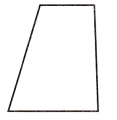So,
The types of quadrilaterals you can find in the seating chart of the field are: General Quadrilateral and Rectangle

Math Talk
Mathematical Processes
Explain how trapezoids and parallelograms are different?
A trapezoid has one pair of parallel sides and a parallelogram has two pairs of parallel sides. A trapezoid has at least one pair of parallel sides, but it can also have another.

Activity

Materials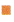quadrilateralsscissors

You can use a Venn diagram to sort quadrilaterals and find out how they are related.

• Draw the diagram below on your MathBoard.
• Cut out the quadrilaterals and sort them into the Venn diagram.
• Record your work by drawing each figure you have placed in the Venn diagram below.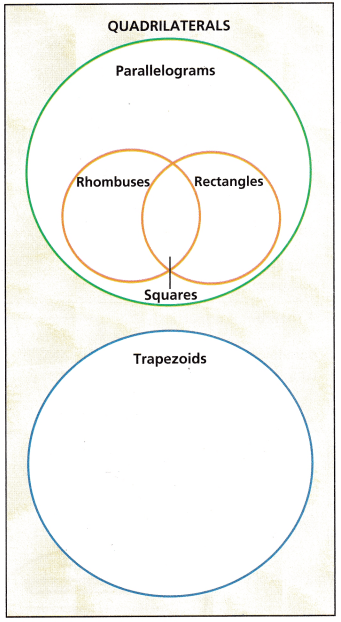Complete the sentences by writing always, sometimes, or never.

A rhombus is “Sometimes” a square.
A parallelogram is _”Sometimes” a rectangle.
A rhombus is “Always” a parallelogram.
A trapezoid is “Sometimes” a parallelogram.
A square is “Always” a rhombus.

Question 1.
Explain why the circle for parallelograms does not intersect the circle for trapezoids.
We know that,
The “Parallelogram” has 2 pairs of parallel sides and the “trapezium” has one pair of parallel sides only
Hence, from the above,
We can conclude that
The circle for parallelograms does not intersect the circle for trapezoids

Question 2.
Draw a quadrilateral with four pairs of perpendicular sides and four congruent sides.
It is given that
Draw a quadrilateral with four pairs of perpendicular sides and four congruent sides.
Hence, from the above information,
The representation of the quadrilateral with the given specifications is: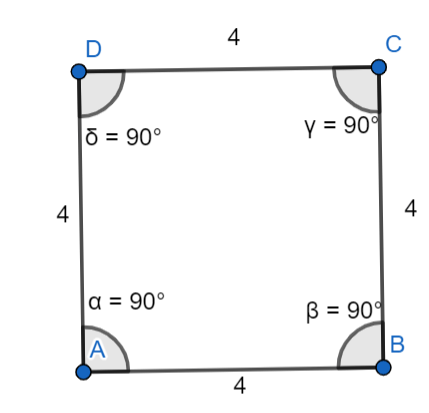Share and Show

Question 1.a. Measure the sides. Are any of the sides congruent? ___
Mark any congruent sides.
In quadrilateral ABCD, there are congruent sides
Hence,
The representation of the congruent sides in the quadrilateral ABCD is: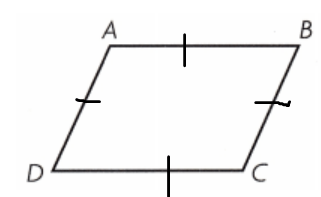b. How many right angles, if any, does the quadrilateral have? ____
The representation of quadrilateral ABCD with its congruent sides is:Now,
We can observe that
There are no angles that have the angle measure of 90°
Hence, from the above,
We can conclude that
There are no right angles in a quadrilateral ABCD

c. How many pairs of parallel sides, if any, does the quadrilateral have?
The representation of quadrilateral ABCD with its congruent sides is:Now,
We can observe that
There are 2 pairs of parallel sides in the quadrilateral ABCD
Hence, from the above,
We can conclude that
The number of parallel sides the quadrilateral ABCD has is: 2 pairs
So,
The quadrilateral ABCD is a “Parallelogram”

Math Talk
Mathematical Processes
Can the parallel sides of a trapezoid be the same length? Explain your answer.
We know that,
In a trapezoid, there is only 1 pair of parallel sides i.e., the sides that have the base angles
In an isosceles trapezoid, two opposite sides (the bases) are parallel, and the two other sides (the legs) are of equal length (properties shared with the parallelogram).

Classify the quadrilateral in as many ways as possible. Write quadrilateral, parallelogram, rectangle, rhombus, square, or trapezoid.

Question 2.Now,
We can observe that
There are 4 pairs of congruent sides and all the congruent angles that are perpendicular
Hence, from the above,
We can conclude that
The shape of the given quadrilateral is: Rectangle

Question 3.Now,
We can observe that
The shape is: Flat-based
There is only 1 pair of parallel sides
Hence, from the above,
We can conclude that
The shape of the given quadrilateral is: Trapezium (or) Trapezoid

Problem Solving

Solve the problems.

Question 4.
H.O.T. What’s the Error? A quadrilateral has exactly 3 congruent sides. Davis claims that the figure must be a rectangle. Why is his claim incorrect? Use diagrams to explain your answer.It is given that
A quadrilateral has exactly 3 congruent sides. Davis claims that the figure must be a rectangle.
Now,
We know that,
A triangle has 3 sides
A rectangle has 4 pairs of parallel sides with 4 congruent angles of 90°
Hence, from the above,
We can conclude that
The error in Davis’ claim is:
A rectangle has 4 pairs of parallel sides with 4 congruent angles of 90°Question 5.
Multi-Step I am a figure with four sides. I can be placed in the following categories: quadrilateral, parallelogram, rectangle, rhombus, and square. Draw me. Explain why I fit into each category.
It is given that
I am a figure with four sides. I can be placed in the following categories: quadrilateral, parallelogram, rectangle, rhombus, and square
Now,
We know that,
A “Quadrilateral” is a shape that has 4 sides
Now,
The representation of the given figures is: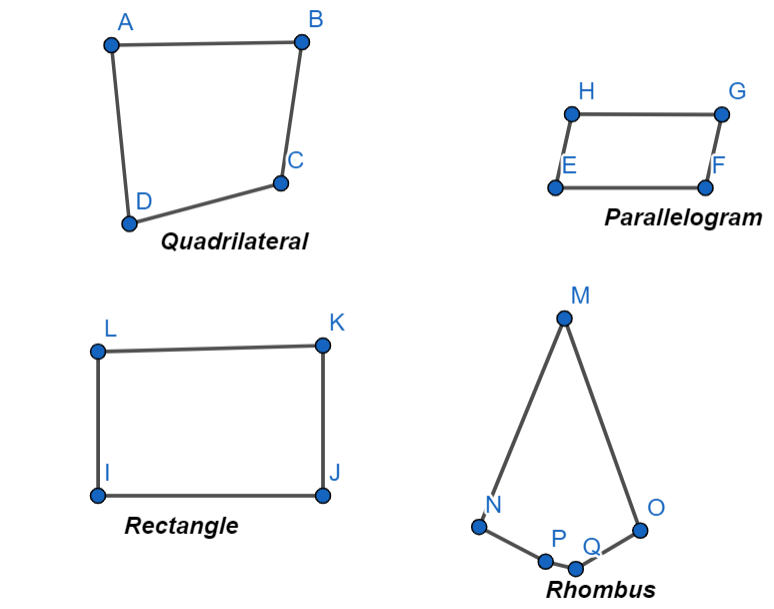Hence, from the above,
We can conclude that
A “Quadrilateral” is a shape that has 4 sides

Question 6.
Rita only uses parallelograms in her design. Which of the following shapes can she use?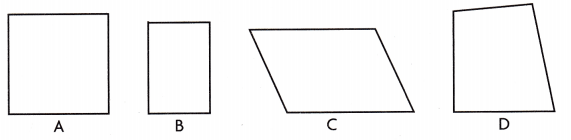(A) B, C, D only
(B) A, B, C only
(C) A and D only
(D) B and C only
It is given that
Rita only uses parallelograms in her design
Now,
We know that,
A “Parallelogram” will sometimes be a “rectangle”
Hence, from the above,
We can conclude that
The shapes that Rita can use is:Question 7.
Which statement is true about squares and rhombuses?
(A) A square is never a rhombus.
(B) A rhombus is never a square.
(C) A square is always a rhombus.
(D) A rhombus is always a square.
We know that,
A “Square” is always a “Rhombus”
A “Rhombus” is sometimes a “Square”
Hence, from the above,
We can conclude that
The statement that is true about squares and rhombuses is:Question 8.
Multi-Step A quadrilateral has two pairs of parallel sides. It also has four congruent sides. What might the shape be?
(A) square or rhombus
(B) rectangle or trapezoid
(C) square or trapezoid
(D) trapezoid or parallelogram
It is given that
A quadrilateral has two pairs of parallel sides. It also has four congruent sides
Hence, from the above,
We can conclude that
The shapes might be:Texas Test Prep

Question 9.
A quadrilateral has exactly 1 pair of parallel sides and no congruent sides. What type of quadrilateral is it?
(A) rectangle
(B) rhombus
(C) parallelogram
(D) trapezoid
It is given that
A quadrilateral has exactly 1 pair of parallel sides and no congruent sides
Hence, from the above,
We can conclude that
The type of quadrilateral with the given information is: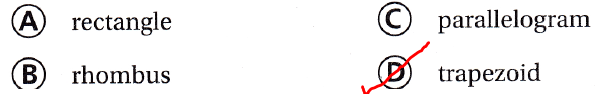### Texas Go Math Grade 5 Lesson 11.3 Homework and Practice Answer Key

Classify the quadrilateral in as many ways as possible. Write quadrilateral, parallelogram, rectangle, rhombus, square, or trapezoid.

Question 1.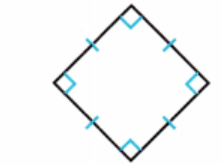Now,
We can observe that
All the sides are congruent
All the angles are congruent
Hence, from the above,
We can conclude that

Question 2.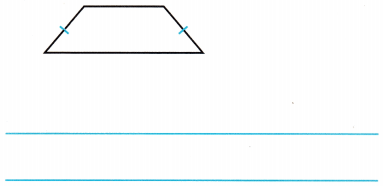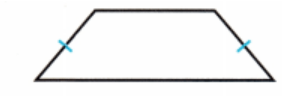Now,
We can observe that
There is only 1 pair of parallel sides and no congruent sides
Hence, from the above,
We can conclude that
The given quadrilateral is: Trapezoid (or) trapezium

Question 3.Now,
We can observe that
There are no congruent side lengths and angle measures
Hence, from the above,
We can conclude that

Question 4.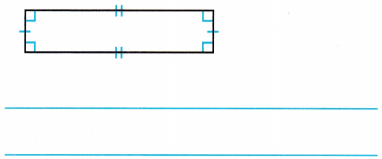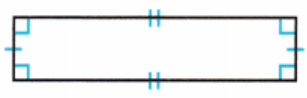Now,
We can observe that
All the angle measures are congruent and have an angle of 90°
There are 2 pairs of parallel sides and 4 pairs of congruent sides
Hence, from the above,
We can conclude that

Question 5.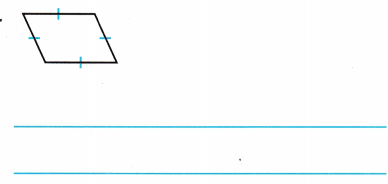Now,
We can observe that
There are 2 pairs of parallel sides
There are no congruent angle measures
Hence, from the above,
We can conclude that

Question 6.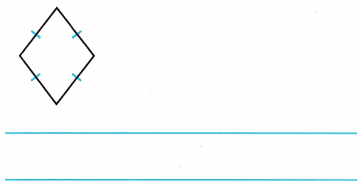Now,
We can observe that
There are 2 pairs of parallel sides and 4 pairs of congruent sides
There are no congruent angle measures
Hence, from the above,
We can conclude that

Problem Solving

Question 7.
Brooke draws quadrilaterals on a canvas in her art class. Is it possible for Brooke to draw a parallelogram that is not a rectangle? Explain.
It is given that
Brooke draws quadrilaterals on a canvas in her art class
Now,
We know that,
There are 3 types of parallelograms. They are:
a. Rhombus
b. Rectangle
c. Square
Now,
Brooke can draw quadrilaterals on a canvas that is not a rectangle i.e., Brooke can draw squares and rhombuses on a canvas
Hence, from the above,
We can conclude that
It is possible for Brooke to draw a parallelogram that is not a rectangle

Question 8.
Is it possible for Brooke to draw a square and a rhombus that are congruent? Explain.
We know that,
There are 3 types of parallelograms. They are:
a. Rhombus
b. Rectangle
c. Square
Now,
We know that,
A square is always a rhombus but a rhombus will not always be a square
Hence, from the above,
We can conclude that
It may be possible for Brooke to draw a square and a rhombus that are congruent

Texas Test Prep

Lesson Check

Question 9.
Which figures have perpendicular sides?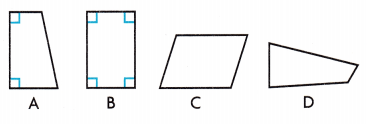(A) A and B only
(B) A and C only
(C) B and D only
(D) C and D only
We know that,
The “Perpendicular side” is nothing but a side with an angle of 90°
Hence, from the above,
We can conclude that
The figures that have perpendicular sides are:Question 10.
Which statement is true about the two figures below?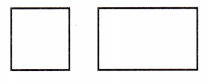(A) They have a different number of right angles.
(B) They are not congruent.
(C) They are rhombuses.
(D) They are squares.
The given figures are:Now,
From the given figures,
We can observe that
The first and second figures are Square and rectangle respectively
Now,
We know that,
In a rectangle, 2 pairs of parallel sides and 4 congruent angles are present
In a square, 4 pairs of congruent sides and angles are present
Hence, from the above,
We can conclude that
The statement that is true about the given two figures is: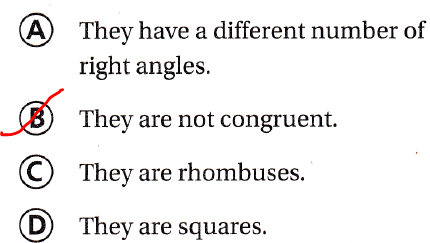Question 11.
Which of the following is NOT a parallelogram?
(A) rhombus
(B) square
(C) trapezoid
(D) rectangle
We know that,
There are 3 types of parallelograms. They are:
a. Rhombus
b. Rectangle
c. Square
Hence, from the above,
We can conclude that
The quadrilateral that is not a parallelogram is: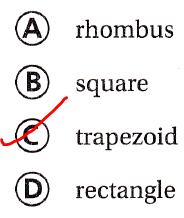Question 12.
Which of the following is a regular polygon?
(A) parallelogram
(B) rectangle
(C) rhombus
(D) square
We know that,
A “Regular Polygon” is a polygon that has congruent sides and angle measures
Hence, from the above,
We can conclude that
The regular polygon is:Question 13.
Multi-Step Becka draws two different quadrilaterals. Both quadrilaterals have two pairs of parallel sides. Only one quadrilateral has four right angles. What might the quadrilaterals be?
(A) square and parallelogram
(B) rectangle and square
(C) rectangle and trapezoid
(D) parallelogram and trapezoid
It is given that
Becka draws two different quadrilaterals. Both quadrilaterals have two pairs of parallel sides. Only one quadrilateral has four right angles
Now,
From the given information,
We can observe that
The first quadrilateral will be a “Parallelogram”
The second quadrilateral will be a “Rectangle” or a “Square”
Hence, from the above,
We can conclude thatQuestion 14.
Multi-Step Tim cuts out paper quadrilaterals like the one below to use in an art project.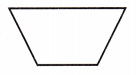If he cuts the quadrilateral in half, which quadrilaterals result from the cut?
(A) two rhombuses
(B) two parallelograms
(C) two trapezoids
(D) a trapezoid and a parallelogram
It is given that
Tim cuts out paper quadrilaterals like the one below to use in an art project.
Now,
The given figure is:Now,
From the given figure,
We can observe that
When the parallelogram is cut into 2 halves,
We can say that 2 parallelograms will be formed
Hence from the above,
We can conclude that
The quadrilateral that results from the cut is:Scroll to Top